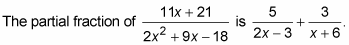##### Trigonometry Workbook For DummiesA process called partial fractions takes one fraction and expresses it as the sum or difference of two other fractions. In calculus, this process is useful before you integrate a function. Because integration is so much easier when the degree of a rational function is 1 in the denominator, partial fraction decomposition is a useful tool for you.

The process of decomposing partial fractions requires you to separate the fraction into two (or sometimes more) disjointed fractions with variables (usually A, B, C, and so on) standing in as placeholders in the numerator. Then you can set up a system of equations to solve for these variables. For instance, you must follow these steps to write the partial fraction decomposition of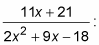1. Factor the denominator and rewrite it as A over one factor and B over the other.

You do this because you want to break the fraction into two. The process unfolds as follows: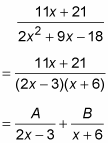2. Multiply every term you’ve created by the factored denominator and then cancel.

You’ll multiply a total of three times in this example: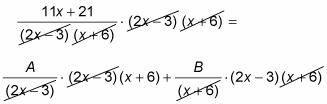This equals 11x + 21 = A(x + 6) + B(2x – 3).

3. Distribute A and B.

This gives you 11x + 21 = Ax + 6A + 2Bx – 3B.

4. On the right side of the equation only, put all terms with an x together and all terms without it together.

Rearranging gives you 11x + 21 = Ax + 2Bx + 6A – 3B.

5. Factor out the x from the terms on the right side.

You now have 11x + 21 = (A + 2B)x + 6A – 3B.

6. Create a system out of this equation by pairing up terms.

For an equation to work, everything must be in balance. Because of this fact, the coefficients of x must be equal and the constants must be equal. If the coefficient of x is 11 on the left and A + 2B on the right, you can say that 11 = A + 2B is one equation. Constants are the terms with no variable, and in this case, the constant on the left is 21. On the right side, 6A – 3B is the constant (because there is no variable attached) and so 21 = 6A – 3B.

7. Solve the system, using either substitution or elimination.

In this example, you use elimination in this system. If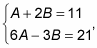you can multiply the top equation by –6 and then add to eliminate and solve. You find that A = 5 and B = 3.

8. Write the solution as the sum of two fractions.